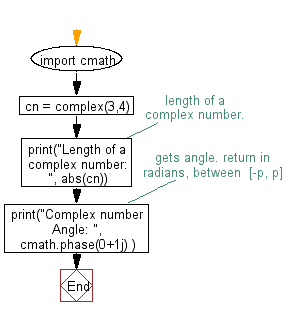﻿ Python Math: Get the length and angle of a complex number - w3resource# Python Math: Get the length and angle of a complex number

## Python Math: Exercise-34 with Solution

Write a Python program to get the length and the angle of a complex number.

Sample Solution:-

Python Code:

``````import cmath
cn = complex(3,4)
#length of a complex number.
print("Length of a complex number: ", abs(cn))
# gets angle. return in radians, between  [-π, π]
print("Complex number Angle: ",cmath.phase(0+1j) )
```
```

Sample Output:

```Length of a complex number:  5.0
Complex number Angle:  1.5707963267948966
```

Flowchart:Python Code Editor:

Have another way to solve this solution? Contribute your code (and comments) through Disqus.

What is the difficulty level of this exercise?

Test your Programming skills with w3resource's quiz.

﻿

```>>> students = [{'name': 'John', 'score': 98}, {'name': 'Mike', 'score': 94}, {'name': 'Jennifer', 'score': 99}]